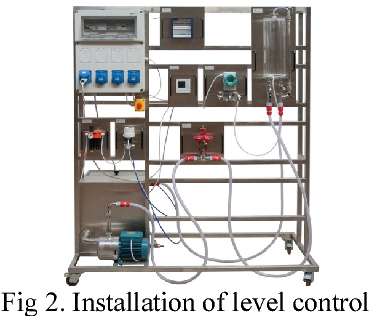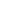### الصفحاتم4 القياسات والتحكم مرحلة رابعة

# PI Level Control مختبر القياسات و التحكم

EXP.NO.4

PI  Level Control

التجربة كامله مع الحسابات العمليه والمخططات وحل اسئلة المناقشه

Objectives

1.     in the following practical's ,you will investigate PI and PID control ,comparing the two and demonstrating any drawbacks associated with them.

2.     To learn about proportional plus integral PI control when used to control the level in the tank on the BPR.

3.     To learn the limitations of  PI control in particular situations ,providing the reason why another control method is required PID.

4.     To learn about full three PID control for controlling the level in the tank.

Proportional and Integral PI control

The major problem with proportional control , as explored is the inherent offset produced by the controller .the control effort needed to correct an error is directly proportional to that error and so the minimum error possible is finite. The way to remove this error is to use a control action that will produce a control effort for zero error. This is done by introducing an extra component into the control effort that is the integral of the error. This continuous to change until the error is zero.

Integral control

The amount of integral action is controlled by constant  which is the reset time. the control effort to the process is now described by

Uc=UP+Ur

Where

: the proportional term

: the reset (integral) term

From the previous assignments , you will already have met the expression for the proportional term

Up=Ke

The reset term is described by :

Ur=(k/Tr ) ∫ e dt

Which shows the position of the reset time constant  Tr

The control effort can now be determined by the following expression

Uc=k[(1/Tr ) ∫e dt]

This describes the action of an automatic reset controller

The diagram above is the pervious proportional control example where

Uc=ke

The diagram below shows the new control effort arrangement to produce PI control. Now you can see the two distinct elements of  Uc:Ur is the reset term and  Up is the proportional term

The reset time constant TR is a very important variable as it controls the contribution of the integral constant to the control effort over a given length of time

Calculations and graphics

A-   Proportional control only : P-control

I = 1

 case PB e.s.s Ts (sec) Tr (sec) TP (sec) Mp 1 1 1.0 50 24 35 10.6 2 5 1.5 60 23 35 9.00 3 10 2.0 59 28 38 7.50 4 15 3.5 61 28 40 7.80 5 20 4.0 63 42 51 6.60

B-Proportional – integral control

PB = 5

 case Tr e.s.s Ts (sec) Tr (sec) TP(sec) Mp 1 5 1.00 48 56 64 1 2 10 0.50 50 47 53 1 3 15 0.25 68 29 38 6 4 20 1.50 54 26 35 8

DISCUSSION

1.     How does proportional band effect the response time of the controller  ( consider what PB is and how it influences the control effort.

Ans . when the proportional increasing the  offset  increasing to suitable  value after this the increasing in PB produced decreasing in offset but the system become unstable.

2.     Recall the simple equations met in earlier theory sections and explain how PB and  can be treated off against each other produce a desired control action.

Ans. The overall TRANSFER FUNCTIOIN  of PID controller is

C(S)=KP+(KI/S)+KD S =(KD S^2+KP S+KI)/S

Where

KP : proportional gain

kI : integral gain

KD : derivative gain

Recall this equation

U=KP e+KI ∫edt+KD  (de/dt )

 Response Rise time overshoot Settling time s-s error KP Decrease increase NT Decrease kI Decrease increase increase Eliminate KD NT Decrease Decrease NT

3.     Why must the integral action be large if PB is large (explain each step linking these two parameters

Ans. When increasing the integral action the offset error is decrease until this error become error.مهندس ميكانيك
هدفي هو التطوير من ذاتي ثم الاستفادة ثم تطوير غيري